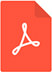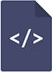# Comparison between FLC and PID controller for speed control of DC motorPDF Full Text

# Abstract

The conventional PID controller is very good when all parameters of the system are Known, that’s mean the system is linear, but when the system is nonlinear because of the friction, the saturation, the disturbance, or the load the dynamic model of the system will be the change, in this case, the conventional PID controller is not good, therefore we used the FLC because it is considered as intelligent controller and it is very suitable when parameter changed or not known, FLC does not need to now the all dynamic model of the system, that’s mean FLC is good with nonlinear system. In this paper, we presented the modeling of DC Motor, and how to compute the full dynamic model of DC Motor, after that we built a subsystem for DC Motor using MATLAB / Simulink, then we applied the PID controller and fuzzy logic controller for controlling the speed of DC Motor, the parameters of PID controller proportional (P), integral (I), derivative (D), tuned by using PID tuning tool in Simulink, we designed FLC for speed control, then we compared the simulation results between PID and FLC, in characteristics rise time, settling time, steady-state error and overshoot. From the simulation results and table compared we found that the performance of FLC is better than the performance of the PID controller in terms of speed control in case of no disturbance or load and with disturbance or load.

# Keywords

Fuzzy logic controller (FLC), PID controller, DC motor, speed control